## Textbook Answers – Changing the Subject

July 19, 2016

Workout

Question 1:

(a)   y = c – w          (b)   y = m + p         (c)   y = s – m

(d)   y = n + 2g       (e)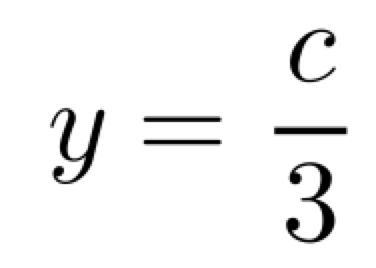(f)(g)  y = cw              (h)  y = 2ac              (i)   y = a – p

(j)  y = c + k          (k)  y = √s                 (l)  y = ∛x

(m)  y = g²           (n)(o)  y = n – t

(p)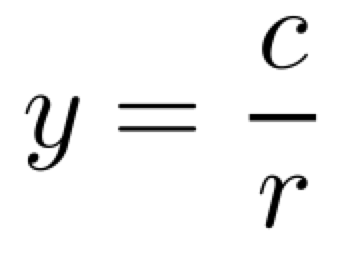(q)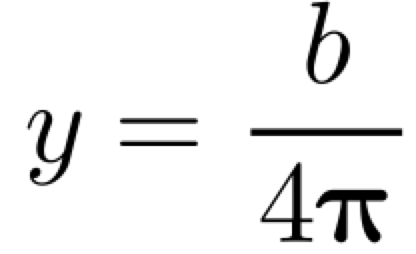(r)   y = c + r – 7t

(s)(t)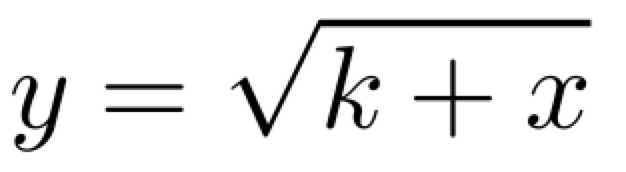(u)Question 2:

(a)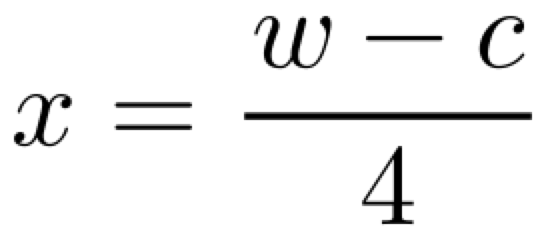(b)(c)(d)(e)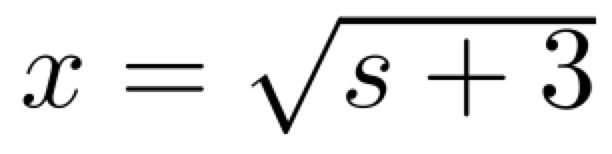(f)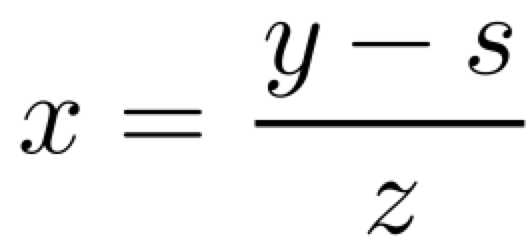(g)   x = n(w – 2)          (h)  x = 6(w + 5)     (i)  x = ch – 3

(j)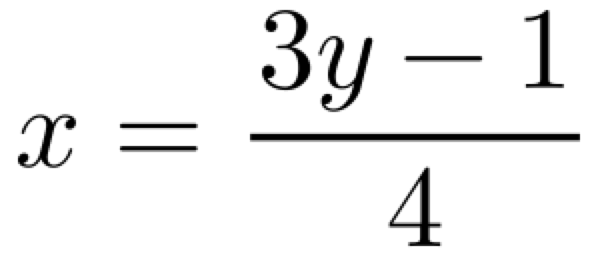(k)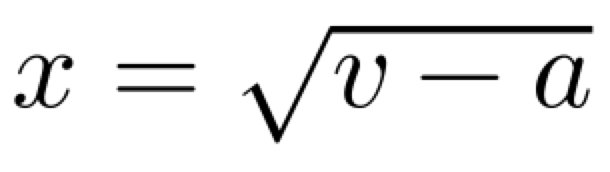(l)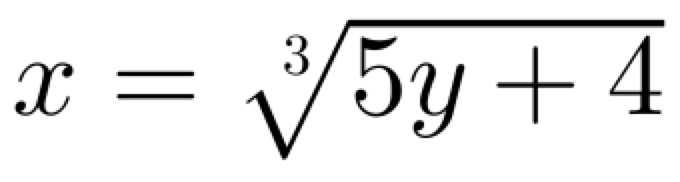(m)  x = 2cm – t         (n)  x = 3uz – w       (o)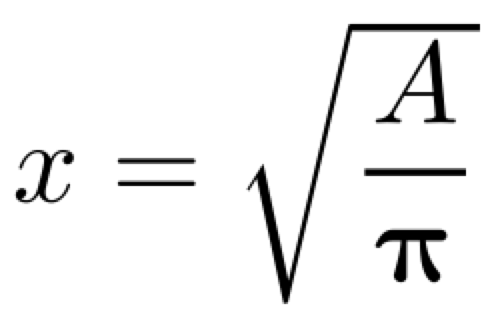(p)(q)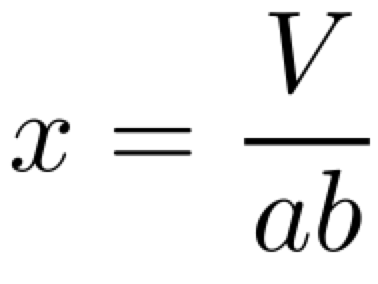(r)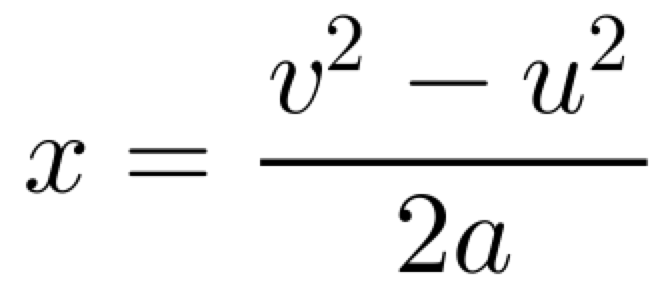(s)(t)(u)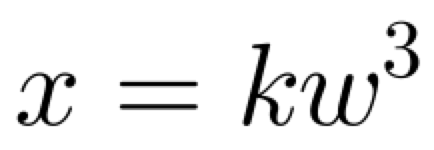Question 3:

(a)(b)(c)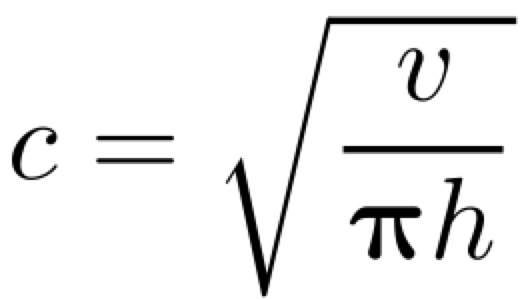Apply

Question 1: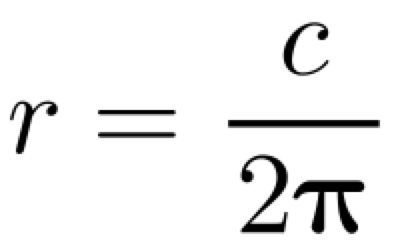Question 2:Question 3(a):  the a should be subtracted from both sides before square rooting

Question 3(b):  the first step is correct, but then both sides should be multiplied by 4.